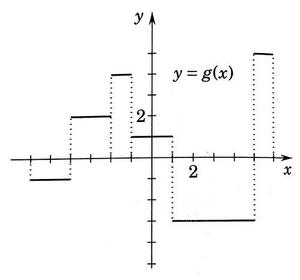# Step function integral

Gold Member
The problem
I want to calculate ## \int^6_{-6} \frac{g(x)}{2+g(x)} \ dx ## for the step function below.The attempt
I started with rewriting the function as with the help of long-division
## \int^6_{-6} \frac{g(x)}{2+g(x)} \ dx = \int^6_{-6} 1 \ dx - 2\int^6_{-6} \frac{1}{g(x)+2} \ dx##

I know that ##\int^6_{-6} 1 \ dx = 12## but thats about it. I am not sure how I should continue.

And here is where I get stuck.

Last edited:

## Answers and Replies

Charles Link
Homework Helper
Gold Member
2020 Award
If you look closely at ## g(x) ##, it takes on constant values for various intervals. You need to break up the integral from -6 to 6 into these various segments.

•Rectifier
Mark44
Mentor
The problem
I want to calculate ## \int^6_{-6} \frac{g(x)}{2+g(x)} \ dx ## for the step function below.The attempt
I started with rewriting the function as with the help of long-division
## \int^6_{-6} \frac{g(x)}{2+g(x)} \ dx = \int^6_{-6} 1 \ dx - 2\int^6_{-6} \frac{1}{g(x)+2} \ dx##

I know that ##\int^6_{-6} 1 \ dx = 12## but thats about it. I am not sure how I should continue.

And here is where I get stuck.
This shouldn't be too difficult. On the interval [-6, -4], g(x) = -1, so g(x) + 2 = 1. What is ##\int_{-6}^{-4} \frac 1 1 dx##? Do the same for the other intervals.

•Rectifier and Charles Link
Buzz Bloom
Gold Member
Hi Rectifier:

I suggest breaking the integral into six pieces, one piece for each step. For each piece, g(x) has a specific constant value, so the integrand is a specific constant.

Hope this helps.

Regards,
Buzz

•Rectifier
Gold Member
Thank you for your help, everyone!

•Charles Link